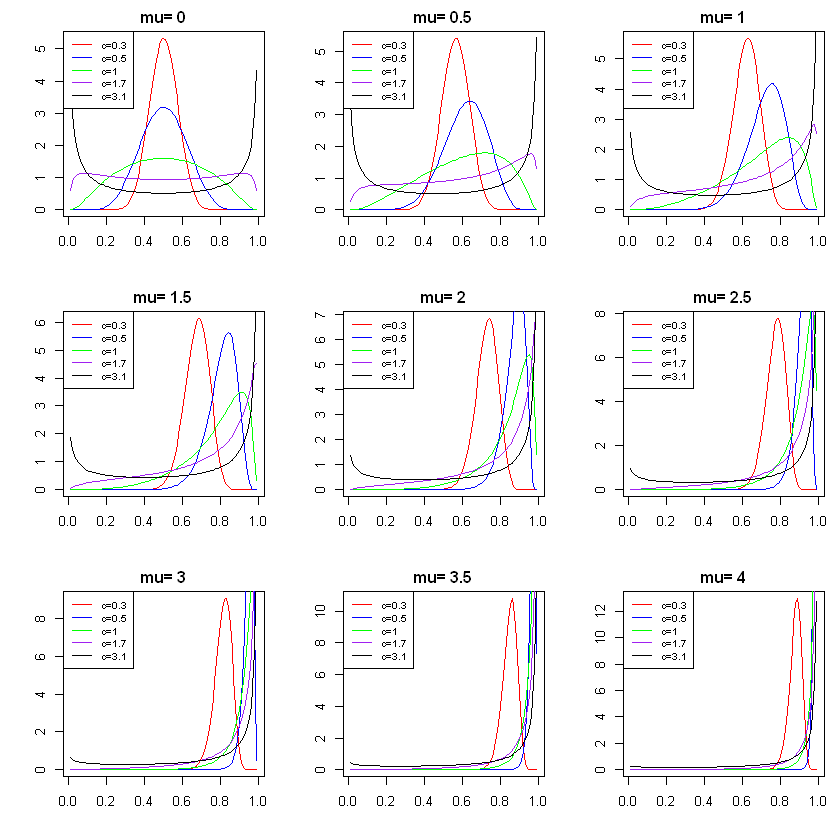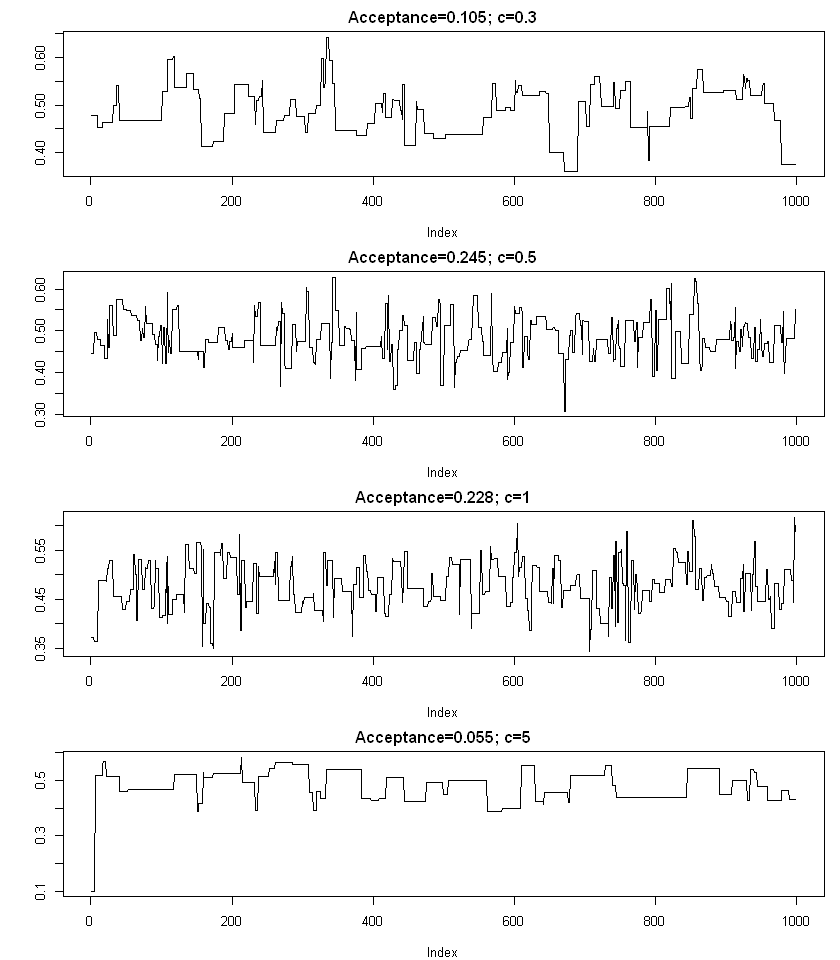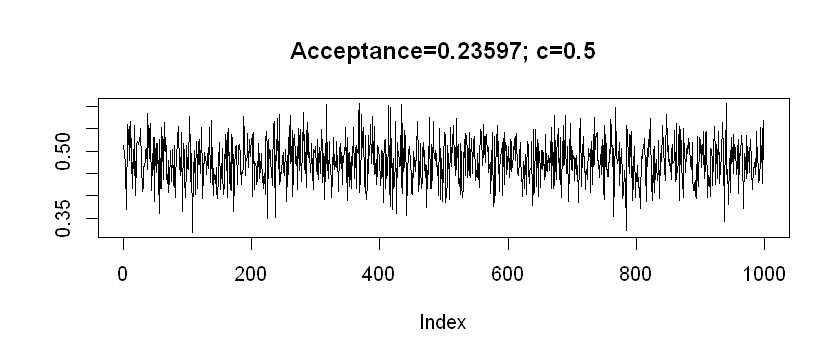# The Metropolis-Hastings Algoriothm I¶

Nov. 15, 2018 $\newcommand{\bs}{\boldsymbol}$ $\newcommand{\argmin}{\underset{\bs{#1}}{\text{arg min}}\,}$ $\newcommand{\argmax}{\underset{\bs{#1}}{\text{arg max}}\,}$ $\newcommand{\tr}{^{\top}}$ $\newcommand{\norm}{\left|\left|\,#1\,\right|\right|}$ $\newcommand{\given}{\,|\,}$ $\DeclareMathOperator{\E}{\mathbb{E}}$ $\newcommand{\blue}{{\color{blue} {#1}}}$ $\newcommand{\red}{{\color{red} {#1}}}$ $\newcommand{\orange}{{\color{orange} {#1}}}$

In the Markov Chain Monte Carlo sampling process, if we can recognize the full conditional distributions of all parameters in the model, we are off to a good start, as block sampling from a known distribution is relatively easy to do. However, not all Gibbs samplers have well recognizable full conditional distributions. To sample from full conditional distributions that are not recognizable, we use the Metropolis-Hastings algorithm.

## The Algorithm¶

Suppose we have a full conditional distribution $p(\theta \given Y)$ where $\theta$, for illustration and simplicity, is assumed to be a single variable parameter, and $Y$ represents data. The Metropolis-Hastings algorithm is stated below.

Algorithm 1 (Metropolis-Hastings)   To draw from $p(\theta \given Y)$,

• Initialize the value $\theta^{(1)}$.
• for $i=1,...,N-1$:
• Generate a value $\theta^*$ from a proposal distribution centered at $\theta^{(i)}$, $$\theta^* \sim J(\theta \given \theta^{(i)})$$ For instance, $J$ could be a normal distribution $N(\theta^{(i)}, c^2)$.
• We set $\theta^{(i+1)} \leftarrow \theta^*$ with the acceptance probability equal to $$\alpha=\min\left\{1, \frac{p(\theta^* \given Y)\,J(\theta^{(i)} \given \theta^*)}{p(\theta^{(i)} \given Y)\,J(\theta^* \given \theta^{(i)}) }\right\}.\tag{1}$$

If the proposal distribution is symmetric, then we have the Metropolis algorithm. If $J$ is symmetric, then $J(\theta^* \given \theta^{(i)}) = J(\theta^{(i)} \given \theta^*)$; thus,

$$\alpha = \min\left\{1, \frac{p(\theta^* \given Y)}{p(\theta^{(i)} \given Y)} \right\}.$$

If the proposal distribution equals the target distribution, i.e., $J(\theta^* \given \theta^{(i)}) = p(\theta^* \given Y)$, then we have the Gibbs sampling, where

$$\alpha=\min\left\{1, \frac{p(\theta^* \given Y)\,p(\theta^{(i)} \given Y)}{p(\theta^{(i)} \given Y)\,p(\theta^* \given Y) }\right\} =1.$$

Choosing the variance of the proposal distribution is important. Roughly speaking, if the variance of $J$ is too large, then the newly generated value $\theta^*$ will have a large variability, leading to a low acceptance probability. This means that the chain may be "stuck" at a particular value for a long time before moving to a different value. The opposite is true when the variance is too small, leading to a high acceptance rate, and a very sticky and correlated Markov Chain. Typically, we want to tune the variance parameter that leads to roughly 35% of acceptance rate.

## Benoulli Example¶

For the simplest case let's assume a Bernoulli data model

$$p(\theta \given \bs{Y}) = \theta^{\sum Y_i} (1-\theta)^{n-\sum Y_i},$$

where $\bs{Y} = (Y_1,..., Y_n)\tr$ is the data consists of i.i.d. binary responses generated below using the truth $\theta=0.5$.

In :
# Generating my data
set.seed(1)
Y <- rbinom(100, 1, 0.5)

# Printing the first 10 data points
print(Y[1:10])

  0 0 1 1 0 1 1 1 1 0


Since $\theta^{(i)} \in (0, 1)$, we want the support of the proposal distribution $J$ to also live in $(0, 1)$. One way to do that is to do a logit transform:

$$U:= \log\frac{\theta}{1-\theta} = \text{logit}(\theta),$$

where $U$ is assumed to follow a normal distribution $N(\theta^{(i)}, c^2)$, and $c$ is a tuning parameter. That is,

$$p_U(u) =\frac{1}{(2\pi c^2)^{1/2}} \text{exp}\left(\frac{-1}{2c^2}(u - \theta^{(i)})^2\right)$$

Letting $g$ denote the logit function, we have that $u = g(\theta)$. The derivative of $g$ is

$$g'(\theta) = \frac{1}{\theta} + \frac{1}{1-\theta} = \frac{1}{\theta(1-\theta)}.$$

Hence by the monotone transformation theorem, the proposal distribution is

\begin{aligned} J(\theta\given \theta^{(i)}) &= p_U(g(\theta)) \left| g'(\theta)\right| \\ &\propto \frac{1}{(2\pi c^2)^{1/2}} \text{exp}\left(\frac{-1}{2c^2}\left(\text{logit}(\theta) - \theta^{(i)}\right)^2\right)\frac{1}{\theta(1-\theta)}. \end{aligned}

This is the logit-normal distribution, denoted by $\theta \sim P(N(\mu, \sigma^2))$ where $\text{logit}(\theta) \sim N(\mu, \sigma^2)$, and $P$ represents the logistic function. The $R$ package logitnorm can be used to sample from this distribution. First let's visualize the distribution for various values of $c$.

In :
library(logitnorm)

# Plotting the density
options(repr.plot.width=7, repr.plot.height=7)
par(mfrow=c(3, 3), mar=c(4, 4, 2, 1))

x <- seq(0.01, 0.99, 0.01)
for (i in 0:8) {
plot(x, dlogitnorm(x, i/4, 0.3), type='l',
xlab='', ylab='', main=paste('mu=',i/2), col='red')
lines(x, dlogitnorm(x, i/2, 0.5), col='blue')
lines(x, dlogitnorm(x, i/2, 1), col='green')
lines(x, dlogitnorm(x, i/2, 1.7), col='purple')
lines(x, dlogitnorm(x, i/2, 3.1), col='black')
legend("topleft", legend=c("c=0.3", "c=0.5", "c=1", "c=1.7", "c=3.1"),
col=c("red", "blue", "green", "purple", "black"), lty=1, cex=0.75)
}Now we are ready to implement the MCMC algorithm for the Bernoulli distribution.

In :
# Define log likelihood of Bernoulli model
dbern.log <- function(theta, Y){
n <- length(Y)
sum(Y)*log(theta) + (n-sum(Y))*log(1-theta)
}

In :
# Metropolis Hastings sampling
Bern.mcmc <- function(theta0, N, c, Y, seed=1, thin=1, plot=TRUE) {
#     parameters
#     ------------------
#     N = number of iterations
#     c = standard deviation for U=logit(theta)
#     Y = binary data vector
set.seed(seed)

# acceptance count (for tuning c)
acc = 0

# Initialize theta and create saving device
theta <- theta0
theta.mcmc <- rep(0, N/thin-1)

for (i in 1:(N-1)){

# Generate a value of U
u <- rnorm(1, theta, c)

# Calculate theta=P(u), where P is the logistic function
theta.s <- 1/(1+exp(-u))

# Compute acceptance probability (use log for numerical stability)
top <- dbern.log(theta.s, Y) + dlogitnorm(theta, theta.s, c, log=TRUE)
bottom <- dbern.log(theta, Y) + dlogitnorm(theta.s, theta, c, log=TRUE)
alpha <- min(1, exp(top-bottom))

# MCMC Update
R <- rbinom(1,1,alpha)

if (R==1){
theta <- theta.s
acc <- acc+1
}

# Saving the thinned Markov chain
if (i %% thin == 0){
theta.mcmc[i/thin] = theta
}
}

if (plot==TRUE){
plot(theta.mcmc, type='l', main=paste0('Acceptance=', acc/N, '; c=', c), ylab='')
}

return(theta.mcmc)
}


Let's test our algorithm using the data set $\bs{Y}$ generated earlier. It remains to tune the parameter $c$. We can simply try different values of $c$.

In :
options(repr.plot.width=7, repr.plot.height=8)
par(mfrow=c(4, 1), mar=c(4, 4, 2, 1))

theta1 <- Bern.mcmc(0.1, 1000, 0.3, Y)
theta2 <- Bern.mcmc(0.1, 1000, 0.5, Y)
theta3 <- Bern.mcmc(0.1, 1000, 1, Y)
theta4 <- Bern.mcmc(0.1, 1000, 5, Y)From these plots, we see the importance of variance turning. It appears that $c=0.5$ that produces around 1/4 acceptance rate is the best. Finally we thin the Markov Chain to remove auto-correlation.

In :
options(repr.plot.width=7, repr.plot.height=3)
Theta <- Bern.mcmc(0.1, 100000, 0.5, Y, thin=100)The posterior mean of the Markov Chain can be computed.

In :
mean(Theta)

0.478182355794278